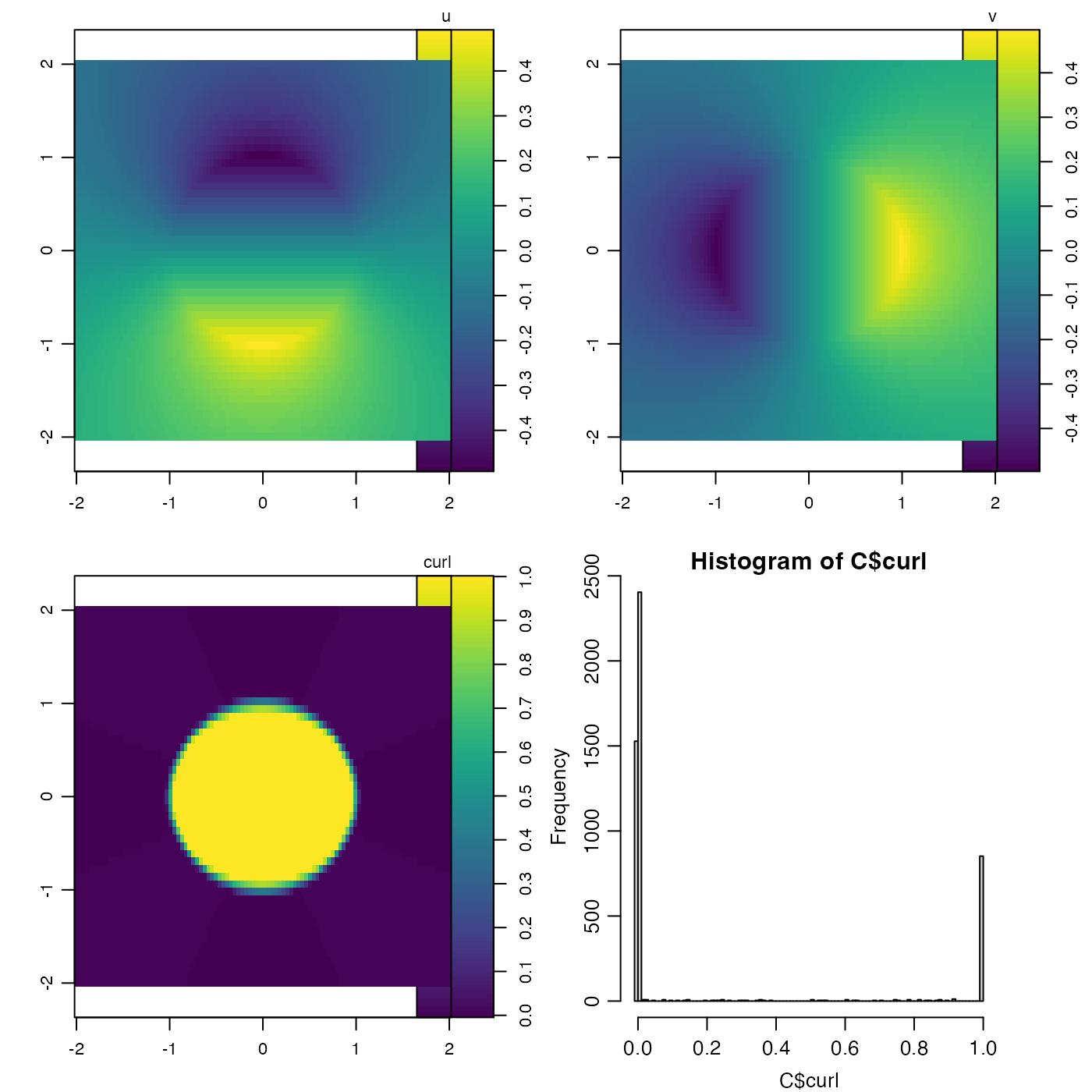Calculate the z component of the curl of an x-y vector field.

curl(u, v, x, y, geographical = FALSE, method = 1)

## Arguments

u matrix containing the 'x' component of a vector field matrix containing the 'y' component of a vector field the x values for the matrices, a vector of length equal to the number of rows in u and v. the y values for the matrices, a vector of length equal to the number of cols in u and v. logical value indicating whether x and y are longitude and latitude, in which case spherical trigonometry is used. A number indicating the method to be used to calculate the first-difference approximations to the derivatives. See “Details”.

## Value

A list containing vectors x and y, along with matrix curl. See “Details” for the lengths and dimensions, for various values of method.

## Details

The computed component of the curl is defined by $$\partial$$$$v/\partial x - \partial u/\partial y$$ and the estimate is made using first-difference approximations to the derivatives. Two methods are provided, selected by the value of method.

• For method=1, a centred-difference, 5-point stencil is used in the interior of the domain. For example, $$\partial v/\partial x$$ is given by the ratio of $$v_{i+1,j}-v_{i-1,j}$$ to the x extent of the grid cell at index $$j$$. (The cell extents depend on the value of geographical.) Then, the edges are filled in with nearest-neighbour values. Finally, the corners are filled in with the adjacent value along a diagonal. If geographical=TRUE, then x and y are taken to be longitude and latitude in degrees, and the earth shape is approximated as a sphere with radius 6371km. The resultant x and y are identical to the provided values, and the resultant curl is a matrix with dimension identical to that of u.

• For method=2, each interior cell in the grid is considered individually, with derivatives calculated at the cell center. For example, $$\partial v/\partial x$$ is given by the ratio of $$0.5*(v_{i+1,j}+v_{i+1,j+1}) - 0.5*(v_{i,j}+v_{i,j+1})$$ to the average of the x extent of the grid cell at indices $$j$$ and $$j+1$$. (The cell extents depend on the value of geographical.) The returned x and y values are the mid-points of the supplied values. Thus, the returned x and y are shorter than the supplied values by 1 item, and the returned curl matrix dimensions are similarly reduced compared with the dimensions of u and v.

## Development status.

This function is under active development as of December 2014 and is unlikely to be stabilized until February 2015.

Other things relating to vector calculus: grad()

## Examples

library(oce)
## 1. Shear flow with uniform curl.
x <- 1:4
y <- 1:10
u <- outer(x, y, function(x, y) y/2)
v <- outer(x, y, function(x, y) -x/2)
C <- curl(u, v, x, y, FALSE)

## 2. Rankine vortex: constant curl inside circle, zero outside
rankine <- function(x, y)
{
r <- sqrt(x^2 + y^2)
theta <- atan2(y, x)
speed <- ifelse(r < 1, 0.5*r, 0.5/r)
list(u=-speed*sin(theta), v=speed*cos(theta))
}
x <- seq(-2, 2, length.out=100)
y <- seq(-2, 2, length.out=50)
u <- outer(x, y, function(x, y) rankine(x, y)$u) v <- outer(x, y, function(x, y) rankine(x, y)$v)
C <- curl(u, v, x, y, FALSE)
## plot results
par(mfrow=c(2, 2))
imagep(x, y, u, zlab="u", asp=1)
imagep(x, y, v, zlab="v", asp=1)
imagep(x, y, C$curl, zlab="curl", asp=1) hist(C$curl, breaks=100)